Worksheets and No Prep Teaching Resources
Math Worksheets

# Sixth Grade Math Worksheets

Sixth grade is a crucial year for students to have complete understanding of basic skills in the four operations as well as the comprehension of prime numbers and factorization, number theory, algebraic reasoning, and equations. Other resourceful worksheets cover fractions in a deep, yet entertaining way, from addition and subtraction to equivalent fractions, ordering, comparing, and improper fractions. Essential decimals skills, geometry, graphing, and probability are covered in other engaging worksheets. Use the dynamic tool to customize entertaining worksheets for your students as they get ready for higher level mathematics!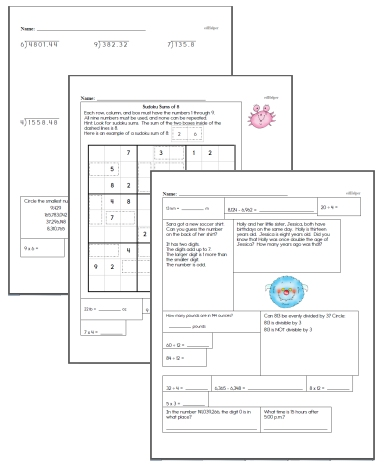Monthly Math Challenge Workbooks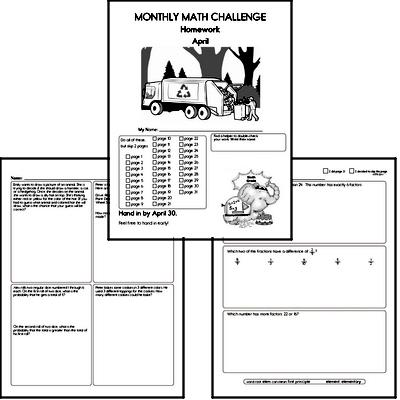6th Grade Math Challenge Book

Mental Math Workbooks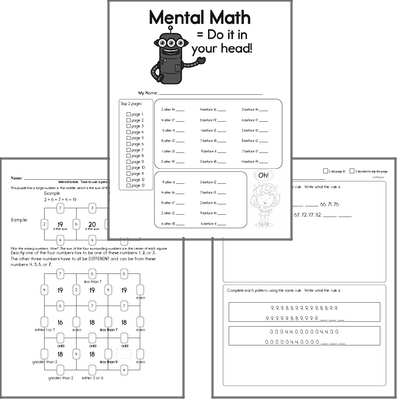Mental Math Workbooks

Sixth Grade Math Worksheets Activity Workbook - No Prep and Not Boring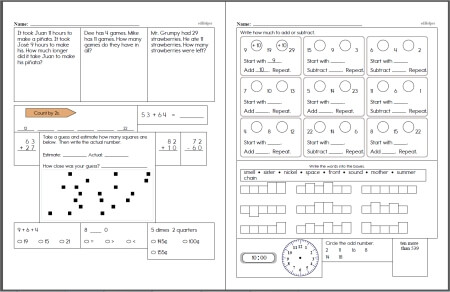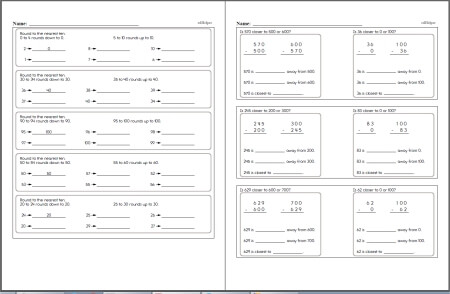Sixth Grade Math Book New Sixth Grade Math Workbook Created Each book contains word problems, mixed math, and other sixth grade review materials. Use it each week for daily practice in your classroom.

Daily Sixth Grade Math and Critical Thinking Practice

Sixth Grade Daily Homework and Practice Pages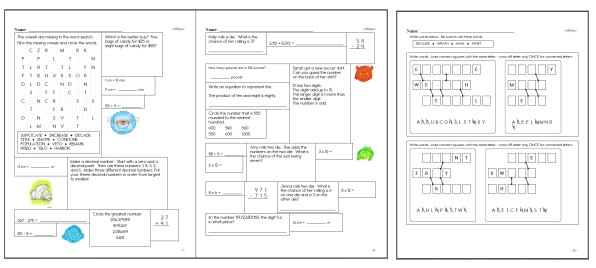Sixth Grade Daily Homework and Practice Pages

Sixth Grade Mixed Review
Level 1: Easiest (First 2 months of school)
Level 2: Next 3 months of school
Level 3: Next 3 months of school
Level 4: Entire Grade (Final months of school)

Weekly Word Problems
Last week's sixth grade math word problems
This week's sixth grade math word problems
Next week's sixth grade math word problems
Daily Review: Customize math review skills with word problems, language arts, and science

edHelperKu Puzzles

Runaway Math Puzzles
 Sixth grade runaway math puzzles Runaway Math Puzzles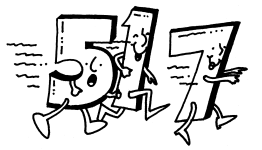Equation Unknowns Puzzle

Build a Mixed Review Worksheet!
For edHelper.com subscribers. - Sign up now by clicking here!

Select the skills to include
Place Value and Basic Operations
Place Value
Subtraction
Multiplication
Division by One-Digit Numbers
Division by Two-Digit Numbers

Number Theory
 Number Theory

Algebraic Reasoning
Algebraic Reasoning
Beginning Algebra

Add and Subtract Fractions and Decimals
 Understanding Fractions Add and Subtract Fractions Understanding Decimals Add and Subtract Decimals

Multiply and Divide Fractions and Decimals
Multiply and Divide Fractions
Multiply Decimals
Divide Decimals

Geometry
 Plane Figures Lines and Angles Area, Circumference, and Perimeter Coordinate Graphing

Measurement
Measurement

Ratios
Ratios

Percents
Percents

Integers
Integers

Probability
Probability

Number of Pages (for each key)
 Select the number of pages: 1 page 2 pages 3 pages 4 pages 5 pages 6 pages

Number of Keys
 Select the number of different printables: 1 key 2 keys 3 keys

Answer key also includes questions

Build a Custom Word Story Worksheet!
For edHelper.com subscribers. - Sign up now by clicking here!
For best results, pick multiple options.
For example, you will not find division problems in the grade 1 material.
Select the skills to include:
 Addition Subtraction Multiplication Division Time Decimals Fractions Percents and Ratios

Select the grade level(s):

 Select the number of word problems: 2 Word Problems 4 Word Problems 6 Word Problems

Number of Keys
 Select the number of different printables: 1 key 2 keys 3 keys

If more than 1 key:
Mix up the questions on each key

(optional)  Enter a class name or header:

Place Value

Place Value
Write each number in standard form.
Write each number in two other ways.
Write the place and the value of the underlined digit.
Fill in the missing number.
Write each number in standard form (information given is mixed up).
Fill in the missing number (not in order of place value).
Write each number in expanded form using exponents.
Write each number in standard form. (includes exponents)
Write each number in standard form. (decimal place value)
Write each decimal in words. (decimal place value)
Write the value of the underlined digit in words. (decimal place value)
Write the value of the underlined digit in words. (whole numbers and decimal place value)
Place Value Mixed Review

Basic Operations
Subtraction
Multiplication
Division

Math Facts

Quick Math Facts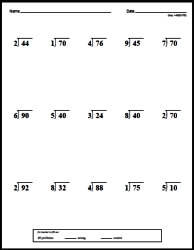Make Quick Math Facts Printable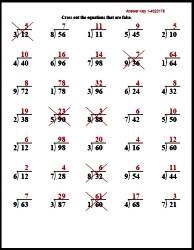2-3 digits column addition
2-4 digits column addition
4-7 digits column addition
2 digits across addition
2-4 digits across addition
4-5 digits across addition
2 digits with 3 numbers column addition
2-3 digits with 3 numbers column addition
2-5 digits with 3 numbers column addition
Column addition: start with 2 digits and increase by 1 digit in each row
Column addition: start with 2-3 digits and increase by 1 digits every 2 rows
Column addition: start with 2-3 digits and increase by 2 digits every 2 rows
Across addition with 3-5 numbers
Fill in the missing numbers: 2 digits
Fill in the missing numbers: 2-3 digits
Fill in the missing numbers: 4-5 digits
Fill in the missing numbers: 2-6 digits
Math word puzzles
Math addition boxes: 3 x 3
Math addition boxes: 4 x 4
Addition Word Problems (Easier)

Number Bonds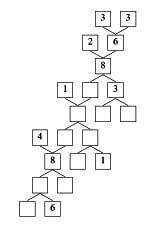Number Bonds

Subtraction
2 digits column subtraction
3 digits column subtraction
2-3 digits column subtraction
4 digits column subtraction
5-6 digits column subtraction
3-5 digits column subtraction
2 digits across subtraction
2-3 digits across subtraction
2-4 digits across subtraction
3-4 digits across subtraction
5-6 digits across subtraction
Column subtraction: start with 2 digits and increase by 1 digit in each row
Column subtraction: start with 2-3 digits and increase by 1 digits every 2 rows
Column subtraction: start with 2-3 digits and increase by 2 digits every 2 rows
Across subtraction with 3-5 numbers
Fill in the missing numbers: 2 digits
Fill in the missing numbers: 2-3 digits
Fill in the missing numbers: 4-5 digits
Fill in the missing numbers: 2-6 digits
Math word puzzles
Math addition boxes: 3 x 3
Math addition boxes: 4 x 4
Subtraction word problems (Easier)
Subtraction word problems
Subtraction Mixed Review

Multiplication
1-2 digits by 1 digit column multiplication
2 digits (and increasing 1 digit every 2 rows) by 1 digit column multiplication
2 digits (and increasing 1 digit every row) by 1 digit column multiplication
Multiples of 10 multiplication (2-5 digits by 1 digit)
2 digits by 2 digits column multiplication
2-3 digits by 2 digits column multiplication
4-5 digits by 2 digits column multiplication
2 digits (and increasing 1 digit every 2 rows) by 2 digit column multiplication
2 digits (and increasing 1 digit every row) by 2 digit column multiplication
1-2 digits by 1 digit across multiplication
3-4 digits by 1 digit across multiplication
1-2 digits by 2 digits across multiplication
Across multiplication with 3-5 numbers
Fill in the missing numbers: 1-2 digits
Fill in the missing numbers: 2 digits
Fill in the missing numbers: 2-3 digits
Math multiplication boxes: 3 x 3 (numbers 1-9)
Math multiplication boxes: 4 x 4 (numbers 1-9)
Math multiplication boxes: 3 x 3 (numbers 4-19)
Multiplication word problems (basic)
Multiplication word problems
Multiplication word problems
2-3 digits by 2-3 digits column multiplication
(extra space for work) 2-3 digits by 2-3 digits column multiplication
Multiples of 10 multiplication (2-5 digits by 1 digit)
(extra space for work) Multiples of 10 multiplication (2-5 digits by 1 digit)
3-4 digits by 3-4 digits column multiplication
(extra space for work) 3-4 digits by 3-4 digits column multiplication
2-5 digits by 2-5 digits column multiplication
(extra space for work) 2-5 digits by 2-5 digits column multiplication
Fill in the missing numbers: 3-4 digits
2-3 digits by 3-5 digits across multiplication
Across multiplication with 3-5 numbers (2 Digits)
Multiplication word problems
Multiplication Mixed Review

Super Silly Squares

Subtraction Squares

Multiplication Squares

Locker Combo Math Learning Center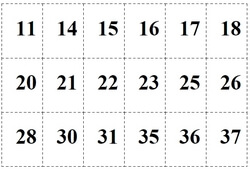Easiest: Open the Locker - Only 1 Number (Grades 4-5) Open the Locker - 2 Numbers in the Locker Combo (Grades 5-6) Open the Locker - 3 Numbers in the Locker Combo (Grades 5-6)

Prime Numbers and Prime Factorization

Number Theory
Prime or composite
Divisibility: complete the table
Divisibility: fill in the missing digit
List all of the factors
Prime factorization
Greatest common factor
Least common multiple
Open-ended number theory questions
Number theory review printable

Number Properties
Number properties
Associative Property
Commutative Property
Distributive Property

Division by One-Digit Numbers
Column Division with Extra Space To Solve Problems - Division by One-Digit Numbers
2 digits by 1 digit column division (no remainders)
2-3 digits by 1 digit column division (no remainders)
4-5 digits by 1 digit column division (no remainders)
5-7 digits by 1 digit column division (no remainders)
2 digits by 1 digit column division
2-3 digits by 1 digit column division
4-5 digits by 1 digit column division
5-7 digits by 1 digit column division
2 digits (and increasing 1 digit every 2 rows) by 1 digit column division
2 digits (and increasing 1 digit every row) by 1 digit column division

Column Division (more problems with less space) - Division by One-Digit Numbers
2 digits by 1 digit column division (no remainders)
2-3 digits by 1 digit column division (no remainders)
4-5 digits by 1 digit column division (no remainders)
5-7 digits by 1 digit column division (no remainders)
2 digits by 1 digit column division
2-3 digits by 1 digit column division
4-5 digits by 1 digit column division
5-7 digits by 1 digit column division
2 digits (and increasing 1 digit every 2 rows) by 1 digit column division
2 digits (and increasing 1 digit every row) by 1 digit column division

Part 2 - Division by One-Digit Numbers
Divisibility: complete the table
2 digits by 1 digit across division
2-3 digits by 1 digit across division
4-5 digits by 1 digit across division
2 digits (and increase by 1 digit every row) by 1 digit across division
Fill in the missing numbers: 2 digits by 1 digit
Fill in the missing numbers: 3 digits by 1 digit
Fill in the missing numbers: 4 digits by 1 digit
Division Word Problems
Division Word Problems
Solve equations: 2 digits by 1 digit
Order of operations: 2-3 digits by 1 digit (no parenthesis)
Order of operations: 2-3 digits by 1 digit
Order of operations: 2-4 digits by 1 digit
One-Digit Numbers Division Mixed Review

Division by Two-Digit Numbers
Column Division with Extra Space To Solve Problems - Division by Two-Digit Numbers
Division by multiples of 10: 3-4 digits by 2 digits (no remainders)
Division by multiples of 10: 5-7 digits by 2 digits (no remainders)
Division by multiples of 10: 3-4 digits by 2 digits
3-4 digits by 2 digits column division (no remainders)
4-5 digits by 2 digits column division (no remainders)
5-6 digits by 2 digits column division (no remainders)
6-7 digits by 2 digits column division (no remainders)
3-4 digits by 2 digits column division
4-5 digits by 2 digits column division
5-6 digits by 2 digits column division
6-7 digits by 2 digits column division
3 digits (and increasing 1 digit every 2 rows) by 2 digits column division
3 digits (and increasing 1 digit every row) by 2 digits column division

Column Division (more problems with less space) - Division by Two-Digit Numbers
Division by multiples of 10: 3-4 digits by 2 digits (no remainders)
Division by multiples of 10: 5-7 digits by 2 digits (no remainders)
Division by multiples of 10: 3-4 digits by 2 digits
3-4 digits by 2 digits column division (no remainders)
4-5 digits by 2 digits column division (no remainders)
5-6 digits by 2 digits column division (no remainders)
6-7 digits by 2 digits column division (no remainders)
3-4 digits by 2 digits column division
4-5 digits by 2 digits column division
5-6 digits by 2 digits column division
6-7 digits by 2 digits column division
3 digits (and increasing 1 digit every 2 rows) by 2 digits column division
3 digits (and increasing 1 digit every row) by 2 digits column division

Part 2 - Division by Two-Digit Numbers
Divisibility: complete the table
3-4 digits by 2 digits across division
4-5 digits by 2 digits across division
5-6 digits by 2 digits across division
3 digits (and increase by 1 digit every row) by 2 digits across division
Fill in the missing numbers: 3 digits by 2 digits
Fill in the missing numbers: 4 digits by 2 digits
Fill in the missing numbers: 5 digits by 2 digits
Division Word Problems
Division Word Problems
Solve equations: 3 digits by 2 digits
Solve equations: 4-5 digits by 2 digits
Order of operations: 2-3 digits by 1 digit
Order of operations: 3 digits by 2 digits
Two-Digit Numbers Division Mixed Review

Algebraic Reasoning
Order of Operations (3-4 numbers, no parenthesis)
Order of operations: simplify
Order of operations: fill in the missing number (1 missing)
Order of operations: fill in the missing operation (1 missing)
Order of operations: fill in all of the numbers
Order of operations: fill in all of the operations
Order of Operations (3-5 digits)
Order of operations: simplify
Order of operations: fill in the missing number (1 missing)
Order of operations: fill in the missing operation (1 missing)
Order of operations: fill in all of the numbers
Order of operations: fill in all of the operations
Algebraic Expressions
Evaluate expressions
More evaluate expressions
Writing algebraic expressions
Solving Equations
Solving subtraction equations
Solving multiplication and division equations
Solving addition, subtraction, multiplication, and division equations
Write an equation for each problem. Then solve the equation.
Functions
Complete the function table (rule and easier inputs given)
Complete the function table (rule and inputs given)
Complete the function table (missing some inputs and some outputs)
Write the rule for the function (all the inputs and outputs given)
Write the rule and fill in missing inputs or outputs
Algebraic Expressions Mixed Review
Algebraic Expressions Mixed Review

Understand Fractions
Circle the equivalent fraction
Write two equivalent fractions
Is each fraction in simplest form?
Write each fraction in simplest form
Equivalent fractions: fill in the missing number (easier)
Equivalent fractions: fill in the missing number
Comparing fractions
Ordering fractions (from least to greatest)
Ordering fractions (from greatest to least)
Part of a number
Problem solving
Circle the equivalent improper fraction
Circle the equivalent mixed number
Write each improper fraction as a mixed number
Write each mixed number as an improper fraction
Open-ended fraction problems
Understand Fractions Mixed Review

Add and Subtract Fractions
Add Fractions with Like Denominators
Add fractions with like denominators (horizontal)
Add fractions with like denominators (vertical)
Add fractions with like denominators: find the value of y
Subtract Fractions with Like Denominators
Subtract fractions with like denominators (horizontal)
Subtract fractions with like denominators (vertical)
Subtract fractions with like denominators: find the value of y
Add and Subtract Fractions with Like Denominators
Add and subtract fractions with like denominators (horizontal)
Add and subtract fractions with like denominators (vertical)
Add and subtract fractions with like denominators: find the value of y
Add and Subtract Fractions with Like Denominators (Mixed Numbers)
Add and subtract fractions with like denominators and mixed numbers (horizontal)
Add and subtract fractions with like denominators and mixed numbers (vertical)
Add and subtract fractions with like denominators and mixed numbers: find the value of y
Add Fractions with Unlike Denominators
Add fractions with unlike denominators (horizontal)
Add fractions with unlike denominators (vertical)
Add fractions with unlike denominators: find the value of y
Subtract Fractions with Unlike Denominators
Subtract fractions with unlike denominators (horizontal)
Subtract fractions with unlike denominators (vertical)
Subtract fractions with unlike denominators: find the value of y
Add and Subtract Fractions with Unlike Denominators
Add and subtract fractions with unlike denominators (horizontal)
Add and subtract fractions with unlike denominators (vertical)
Add and subtract fractions with unlike denominators: find the value of y
Add and Subtract Fractions with Unlike Denominators (Mixed Numbers)
Add and subtract fractions with unlike denominators and mixed numbers (horizontal)
Add and subtract fractions with unlike denominators and mixed numbers (vertical)
Add and subtract fractions with unlike denominators and mixed numbers: find the value of y
Problem Solving
Find parts of a number
Addition and subtraction of fractions
Addition and subtraction of fractions (mixed numbers)
Addition and subtraction of fractions (multi-step)
Addition and subtraction of fractions (more problems)
Review
Add and Subtract Fractions Mixed Review

Understanding Decimals
Tenths
Write each as a decimal (given: graphics, fractions, and word form)
Given graphics, write the decimal (decimals greater than 1)
Write each as a decimal (decimals greater than 1)
Write each as a fraction

Tenths and Hundredths
Hundredths: Write each as a fraction and decimal
Hundredths: Write each as a decimal
Hundredths: Write each as a fraction
Tenths and hundredths: Write each as a decimal
Tenths and hundredths: Write each as a fraction
Hundredths: Write each as a decimal (graphics)
Tenths and hundredths greater than 1: Write each as a decimal
Tenths and hundredths greater than 1: Write each as a fraction
Tenths and hundredths: Write each as a decimal (mix: less than and greater than 1 decimals)
Tenths and hundredths: Write each as a fraction (mix: less than and greater than 1 decimals)
Tenths and hundredths: Compare decimals
Tenths and hundredths: Order decimals
Order decimals (from 6 to 8 numbers each)
Compare decimals and fractions
Order decimals and fractions
Order decimals and fractions (from 7 to 11 numbers each)
Compare decimals and fractions (with numbers greater than 1)
Order decimals and fractions (with numbers greater than 1)
Tenths and Hundredths Mixed Review

Thousandths
Thousandths: Write as a decimal
Thousandths: Write as a fraction
Thousandths: Write as a decimal (decimals greater than 1)
Thousandths: Write as a fraction (decimals greater than 1)

Tenths, Hundredths, and Thousandths
Write as a decimal
Write as a fraction
Compare decimals
Ordering decimals
Compare decimals (including fractions)
Ordering decimals (including fractions)

Open-Ended Questions
Open-ended decimal questions

Review
Mixed Review

Add and Subtract Decimals
Column addition of 2 numbers (up to tenths)
Column addition of 2 numbers (up to hundredths)
Column addition of 2 numbers (up to thousandths)
Money column addition (up to \$99.99)
Money column addition (up to \$99,999.99)
Across addition of 2 numbers (up to hundredths)
Across addition of 2 numbers (up to thousandths)

Subtraction
Column subtraction of 2 numbers (up to tenths)
Column subtraction of 2 numbers (up to hundredths)
Column subtraction of 2 numbers (up to thousandths)
Money column subtraction (up to \$99.99)
Money column subtraction (up to \$99,999.99)
Across subtraction of 2 numbers (up to hundredths)
Across subtraction of 2 numbers (up to thousandths)

Mix of Addition and Subtraction
Column addition and subtraction of 2 numbers (up to tenths)
Column addition and subtraction of 2 numbers (up to hundredths)
Column addition and subtraction of 2 numbers (up to thousandths)
Money column addition and subtraction (up to \$99.99)
Money column addition and subtraction (up to \$99,999.99)
Across addition and subtraction of 2 numbers (up to hundredths)
Across addition and subtraction of 2 numbers (up to thousandths)

Part 2
Column addition of 3 numbers
Order of Operations (no parenthesis)
Order of Operations
Order of Operations (one missing number)
Order of Operations (one missing operation)
Order of Operations (fill in all numbers)
Order of Operations (fill in all operations)
Fill in the missing numbers (up to hundredths)
Fill in the missing numbers (up to thousandths)
Math box
Decimal addition and subtraction word problems
Addition and subtraction of decimals: Mixed Review

Multiply and Divide Fractions
Multiply fractions
Multiply fractions: find the value of y
Multiply fractions with mixed numbers
Multiply fractions with mixed numbers: find the value of y
Divide fractions
Divide fractions: find the value of y
Divide fractions with mixed numbers
Divide fractions with mixed numbers: find the value of y
Multiply and divide fractions
Multiply and divide fractions: find the value of y
Multiply and divide fractions with mixed numbers
Multiply and divide fractions with mixed numbers: find the value of y
Multiply and Divide Fractions Mixed Review

Multiply Decimals
Column Multiplication with Extra Space To Solve Problems
Multiply a decimal (up to hundredths) by a Whole Number
Multiply a decimal (up to ten-thousandths) by a Whole Number
Multiply 2 decimals (up to tenths; numbers are less than 1)
Multiply 2 decimals (up to hundredths; numbers are less than 1)
Multiply 2 decimals (up to thousandths; numbers are less than 1)
Multiply 2 decimals (numbers are from 0 to 999.99)

Column Multiplication (more problems with less space)
Multiply a decimal (up to hundredths) by a Whole Number
Multiply a decimal (up to ten-thousandths) by a Whole Number
Multiply 2 decimals (up to tenths; numbers are less than 1)
Multiply 2 decimals (up to hundredths; numbers are less than 1)
Multiply 2 decimals (up to thousandths; numbers are less than 1)
Multiply 2 decimals (numbers are from 0 to 999.99)

Part 2
Across multiplication: a decimal (up to hundredths) by a Whole Number
Fill in the missing numbers (up to tenths)
Fill in the missing numbers (up to hundredths)
Fill in the missing numbers (up to thousandths)
Order of Operations (no parenthesis; numbers up to 9.9)
Order of Operations (no parenthesis; numbers up to 99.99)
Order of Operations
Order of Operations (one missing number)
Order of Operations (one missing operation)
Order of Operations (fill in all numbers)
Order of Operations (fill in all operations)
Decimal multiplication word problems
Multiplication of decimals: Mixed Review

Divide Decimals
Extra Space To Solve Problems
Divide a decimal (up to hundredths) by a whole number with no remainders
Divide a decimal (up to thousandths) by a whole number with no remainders
Divide a decimal (up to hundredths) by a whole number with remainders (give the remainder)
Add Zeros: Divide a decimal (up to hundredths) by a whole number with no remainders
Add Zeros: Divide a decimal (up to hundredths) by a whole number with no remainders
Add Zeros: Divide a decimal (up to hundredths) by a whole number with no remainders
Add Zeros: Larger numbers; dividing by a whole number
Divide a whole number by a decimal
Divide a decimal by a decimal (up to tenths)
Divide a decimal by a decimal (up to hundredths)

More Problems with Less Space
Divide a decimal (up to hundredths) by a whole number with no remainders
Divide a decimal (up to thousandths) by a whole number with no remainders
Divide a decimal (up to hundredths) by a whole number with remainders (give the remainder)
Add Zeros: Divide a decimal (up to hundredths) by a whole number with no remainders
Add Zeros: Divide a decimal (up to hundredths) by a whole number with no remainders
Add Zeros: Divide a decimal (up to hundredths) by a whole number with no remainders
Add Zeros: Larger numbers; dividing by a whole number
Divide a whole number by a decimal
Divide a decimal by a decimal (up to tenths)
Divide a decimal by a decimal (up to hundredths)

Part 2
Across division: divide a decimal (up to thousandths) by a whole number with no remainders
Order of Operations (no parenthesis, up to 9.9)
Order of Operations (no parenthesis, up to 99.9)
Order of Operations
Order of Operations (one missing number)
Order of Operations (one missing operation)
Order of Operations (fill in all numbers)
Order of Operations (fill in all operations)
Decimal division word problems
Division of decimals: Mixed Review

Beginning Algebra
Evaluate expressions
Writing algebraic expressions
Writing algebraic expressions (compound expressions)
Combining like terms
Solving subtraction equations
Solving addition and subtraction equations (including 3+ terms)
Solving multiplication and division equations
Solving equations (mix)
Beginning Algebra Review Printable

Measurement
Customary units of measure
Customary units of measure (with decimals)
Customary units of measure (with fractions)
Metric units of length
Metric units of mass
Metric units of capacity
Metric units of capacity (with metric tons)
Metric units of capacity (mixed - no metric tons)
Metric units of capacity (mixed)
Unit Conversions (chart)
Unit Conversions (convert quantity)
Adding and subtracting customary units of measure
Adding and subtracting customary units of measure (with fractions)
Ordering Metric units
Measurement review printable

Ratios and Proportions
Write each ratio in three ways (graphics)
Write each ratio in three ways
Complete the ratio table (increasing order)
Complete the ratio table (mixed order)
Write five equivalent ratios for each ratio
Write each ratio in simplest form
Proportions: State whether the ratios are proportional (fractions)
Proportions: State whether the ratios are proportional (mixed ways of writing ratios)
Proportions: Find the missing number (fractions)
Proportions: Find the missing number (mixed ways of writing ratios)
Create a proportion (4 numbers given)
Create a proportion (5 numbers given)
Create a proportion (6 numbers given)
Fill in the blank to complete the proportion
Find the unit rate
Fill in the blank to complete the proportion (more difficult)
Similar figures
Ratios and Proportions Review Printable

Percents
Write the percent of the shaded portion in each grid
Write each fraction as a percent
Write each decimal as a percent
Write each ratio as a percent
Write each fraction, decimal, or ratio as a percent
Write the percent as a fraction
Write the percent as a decimal
Write the percent as a ratio
Algebra: Fill in the missing number
Order 3 numbers from least to greatest
Order 3 numbers from greatest to least
Order 6 numbers from least to greatest
Order 6 numbers from greatest to least
Find 10% of each number
Find the percent of each number (multiples of 5%)
Find the percent of each number
Percent of numbers: Find the missing number
Open-ended questions
Circle graphs
Percents Mixed Review

Plane Figures
Name each figure using letters and symbols
Draw and label an example of each
Classify each pair of lines as parallel, intersecting, or perpendicular
Classify each angle as acute, obtuse, straight, or right
Classify polygons
Classify triangles
Classify triangles according to lengths
Circles
Plane Figures Mixed Review

Lines and Angles
Naming angle pairs (easy)
Naming angle pairs (pictures with 4 angles)
Angle pairs: Find the unknown angle measure
Naming adjacent, complementary, supplementary, and vertical angles
Find the unknown angle measure (pictures with 5 angles, one center)
Naming alternate interior/exterior angles, vertical angles, and corresponding angles
Find the unknown angle measure (traversals with parallel lines)
Lines and Angles Mixed Review

Perimeter

Area

Area and Perimeter
Find the perimeter of each figure
Find the perimeter of each figure using a ruler
Perimeter: Find the value of n
Find the area of each figure
Find the value of n
Find the area and perimeter of each rectangle (no graphics)
Find the perimeter of a complex polygon (composed of 2 rectangles)
Find the perimeter of a complex polygon (composed of 3 rectangles)
Find the perimeter of a complex polygon (composed of 4 rectangles)
Find the area and perimeter of a complex polygon (composed of 2 rectangles)
Find the area and perimeter of a complex polygon (composed of 3 rectangles)
Find the area and perimeter of a complex polygon (composed of 4 rectangles)
Area and Perimeter Mixed Review (Grade 4)

Part 2
Area of a parallelogram (lengths are whole numbers)
Area of a parallelogram (lengths are decimals)
Area of a parallelogram (lengths are fractions)
Area of a triangle (lengths are whole numbers)
Area of a triangle (lengths are decimals)
Area of a triangle (lengths are fractions)
Find the circumference (graphics; lengths are whole numbers)
Find the circumference (graphics; lengths are decimals)
Find the circumference (graphics; lengths are fractions)
Find the circumference (lengths are whole numbers)
Find the circumference (lengths are decimals)
Find the circumference (lengths are fractions)
Area and Perimeter Mixed Review (Grade 5)

Area and Circumference of a Circle
Perfect Squares and Square Roots
Write each perfect square, using the power 2
Write each perfect square as a square root
Find the square root
Find the value of n
Estimate a square root (multiple choice)
Circumference
Find the circumference (graphics; lengths are whole numbers)
Find the circumference (graphics; lengths are decimals)
Find the circumference (graphics; lengths are fractions)
Find the circumference (lengths are whole numbers)
Find the circumference (lengths are decimals)
Find the circumference (lengths are fractions)
Area of a Circle
Area of a circle (graphics; lengths are whole numbers)
Area of a circle (graphics; lengths are decimals)
Area of a circle (graphics; lengths are fractions)
Area of a circle (lengths are whole numbers)
Area of a circle (lengths are decimals)
Area of a circle (lengths are fractions)
Find the area of the partial circles
Review
Area and Circumference of a Circle Mixed Review

Integers
Representing integers
Ordering integers
Addition with 2 numbers
Addition with 2 numbers (fill in the missing number)
Addition with 3-5 numbers
Addition with 3-5 numbers (fill in the missing number)
Subtraction with 2 numbers
Subtraction with 2 numbers (fill in the missing number)
Subtraction with 3-5 numbers
Subtraction with 3-5 numbers (fill in the missing number)
Addition and subtraction (2 numbers)
Addition and subtraction (3-5 numbers)
Addition and subtraction (2 numbers; fill in the missing number)
Addition and subtraction (3-5 numbers; fill in the missing number)
Multiplication
Multiplication (fill in the missing number)
Division
Division (fill in the missing number)
Multiplication and Division (only 2 numbers each)
Addition, subtraction, multiplication, and division mix
Fill in the missing negative signs
Finding absolute value
Comparing integers
Solving addition and subtraction (algebra)
Solving multiplication and division (algebra)
Solving equations (algebra)
Open-ended integer problems
Integers Word Problems Printable
Integers Review Printable

Ordered Pairs and the Coordinate Plane

Coordinate Graphing
Coordinate Plane and Functions
Write the coordinates for each point
Finding ordered pairs
Drawing ordered pairs
Complete the function table
Complete the function table and then graph the function
Make a function from a line
Mixed Review
Transformations
Translations
Reflections
Rotations
Mix of translations, reflections, and rotations
Do multiple translations at once
Review
Coordinate Graphing Mixed Review

Probability
Counting principle
Theoretical probability (write answer as a fraction)
Theoretical probability (write answer as a decimal)
Theoretical probability (write answer as a percent)
Theoretical probability
Compound events (independent events)
Compound events (dependent events)
Compound events
Combinations
Permutations
Combinations and permutations
Probability Review Printable

Test Prep - Concepts
from the test prep section, requires registration
Numeration
Number Concepts
Properties
Fractions and Decimals
Review of Number Concepts

Test Prep - Computation
from the test prep section, requires registration
Subtraction
Multiplication
Division
Review of Computation

Test Prep - Applications
from the test prep section, requires registration
Geometry
Measurement
Problem Solving - Word Problems!
Problem Solving Applications (Charts, etc)

Have a suggestion or would like to leave feedback?